GFG App
Open AppBrowser
Continue

# Newton’s Law Of Universal Gravitation

Newton’s law of Gravitation as the name suggest is given by Sir Isaac Newton. This law helps us to understand the motion of very large bodies in the universe. According to this law, an attractive force always acts between two bodies which have masses. The strength of the force is directly proportional to the mass of the object and is inversely proportional to the square of the distance between them.

## What is the Universal Law of Gravitation?

According to the Law of Universal Gravitation, every point mass in the universe draws every other point mass in the universe by a force pointing in a straight line between their centres of mass, and this force is equal to the masses of the particles and inversely proportional to their difference. From one point to the next, this attractive force often points inward.

This law applies to all objects with weights, no matter how large or small they are. If the difference between two massive objects is very large in comparison to their sizes or if they are spherically symmetric, they may be called point-like masses. In these cases, each object’s mass may be measured by a point mass at its centre of mass.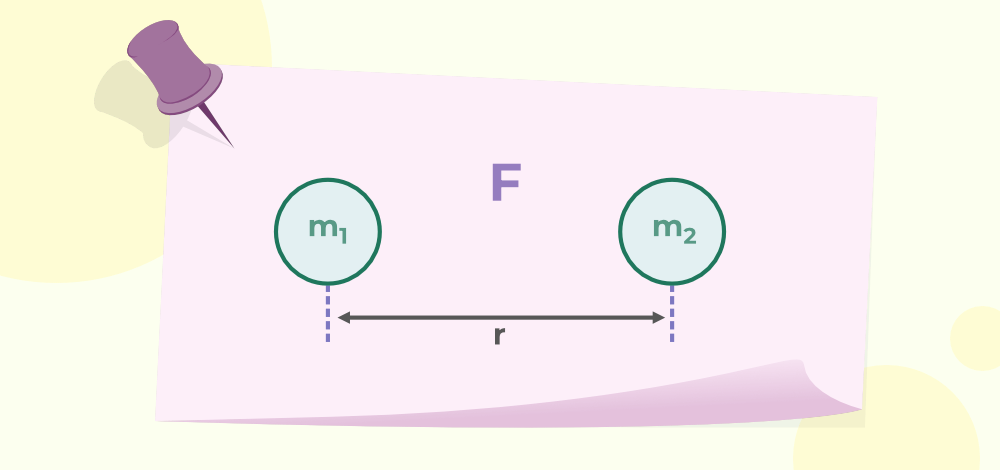## Universal Gravitation Equation

Thus, according to Newton’s law of gravitation, Every particle in the universe attracts every other particle with a force whose magnitude is,

Directly proportional to the product of their masses i.e.

F ∝ (m1 × m2)                 …..(1)

Inversely proportional to the square of the distance between their centres (Also known as Inverse Square law) i.e.

F ∝ (1 / r2)                       ……(2)

On combining equations (1) and (2) it is obtained that,

F ∝ (m1 × m2) / r2            ……(3)

Therefore, from equation (3), it can be said that the gravitational force or force of gravity between two particles or objects is proportional to the product of their masses and the square of the distance between them.

F = G × (m1 × m2) / r2

where,
G is the proportionality constant, also known as the Gravitational constant,
F is the force of gravity,
m1 is the mass of one object,
m2 is the mass of the other object and
r is the distance between those objects.

## Gravitational Constant

Gravitational Constant is a proportionality constant, and it is used in Newton’s Universal Law of Gravitation. It is denoted by G. It can also be defined as a force of attraction between any two unit masses separated by unit distance. From Newton’s Universal Law of Gravitation i.e. from equation (4), the expression for G is,

F = G × (m1 × m2) / r2

G = F × r2 / (m1 × m2)

When,

r =1 m and m1 = m2 = 1 kg then,

G = F

Dimensional Formula for G is [M-1L3T-2].

Its values in SI units is 6.67 × 10-11 Nm2 kg-2 and in the CGS system is 6.67×10-8 dyne cm2 g-2.

## Vector Form of Newton’s Law of Gravitation

The action-reaction pair formed by the gravitational forces acting between the two particles is called the vector form of Newton’s law of gravitation. Consider two point mass bodies A and B of masses m₁ and m₂ placed at distance r apart as shown in the below figure: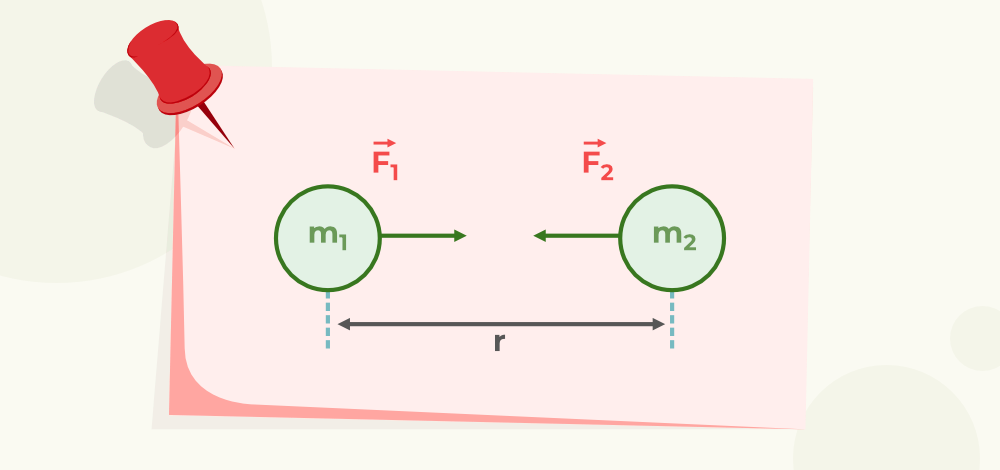Here= Unit vector from A to B= Unit vector from B to A.= Gravitational force exerted on body A by body B.= Gravitational force exerted on body A by body B.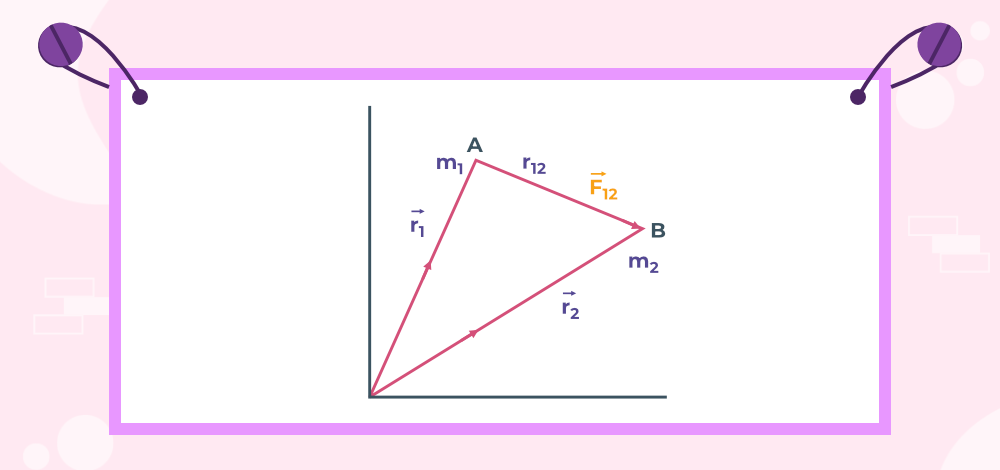From the above figure, it can be seen that the two particles of masses are placed at a distanceTherefore, according to Newton’s law of gravitation, the force applied on m2 by m1 isSimilarly, Gravitational force on m1 by m2We know that| r12 |2 = | r21 |2

Thus,This implies that the above two vectors are equal and opposite to each other which says that gravitational force satisfies Newton’s third law.

Hence, this vector form of Newton’s law of gravitation signifies that the gravitational forces acting between the two particles form the action-reaction pair as shown above.

## Principle of Superposition of Gravitational Forces

The principle of the superposition of gravitational forces states that the total gravitational force on any object is equal to the sum of individual gravitational forces acting on that object. In other words, the net gravitational field at a point is the vector sum of the gravitational fields at that point due to different sources and is given as:whereis the total gravitational force on an object,andare independent forces acting separately on that object.

## Newton’s Law of Gravitation from Kepler’s Law

Suppose a planet of mass m is revolving around the sun of mass M in a nearly circular orbit of radius r, with a constant angular velocity ω. Let T be the time period of revolution of the planet around the sun.

Therefore,

F = mrω2

= mr × (2π/T)2  ……(1)

According to Kepler’s third law, the square of the time period of the planet is proportional to squares of length of semi-major axes and is given as:

T2 ∝ r3

or

T2 = kr3

where k is the proportionality constant, T is the time period of the planet and r is the length of the semi-major axis.

Now apply this in equation (1) as,

F = mr × (4π2 / kr3)

= (4π2 × m) / kr2

But k = 4π2 / GM, substitute this in the above expression as:

F = (G × M × m) / r2

which is the equation of Newton’s law of Gravitation.

## Weight and Gravitational Force

Mass and weight of any substance are two different quantities. Mass is the actual amount of the substance that a substance has, whereas the weight of any substance is the gravitational force exerted on the body by the larger body.

For example, any substance of mass m on the Earth experiences a gravitational force because of the mass of the Earth. The gravitational force on the surface of the earth for mass m is mg where ‘g’ is the gravitational acceleration on earth and its value is 9.8 m/s2. Her, the quantity mg is called the weight of the object.

## Universality of Gravity

The gravitational force explained by Newton is a universal force i.e. it is not only observed on Earth but can be observed anywhere in the universe. According to this law, there is always gravitational force between any two-particle in the universe which is directly proportional to the product of their masses and inversely proportional to the square of their distances.

This law helps scientists to explain planetary motion and the motion of particles in the universe.

## Importance of Gravitational Law

The universal law of Gravitation successfully explained many concepts some of them are,

• Planetary motion is explained by Newton’s Law of Gravitation.
• Interaction among various planets and stars in the universe is explained by Newton’s Law of Gravitation.
• Gravity on the surface of the Earth is explained by Newton’s Law of Gravitation, etc.

## Solved Examples on the Newton’s Law of Gravitation

Example 1: Two bodies of masses 5 kg and 6 kg are placed with their centres 64 m apart. Calculate the initial acceleration of two masses assuming no other forces act on them.

Solution:

Given,

Mass of first body, m1 = 5 kg

Mass of second body, m2 = 6 kg

Distance between two bodies, r = 64 m

Universal Gravitational Constant, G = 6.67 × 10-11 Nm2 kg-2

F = G × (m1 × m2) / r2

Substitute the given values in the above expression as,

F = 6.67 × 10-11 Nm2 kg-2 × (5 kg × 6 kg) / (64 m)2

= 48.85 × 10-14 N

Now, it is known that, the net force on an object is given by,

F = ma

a = F / m

a1 = 48.85 × 10-14 N / 5 kg

= 9.77 × 10-14 m/s2

a2 = 48.85 × 10-14 N / 6 kg

= 8.142 × 10-14 m/s2

Thus, initial accelerations of two bodies are 9.77 × 10-14 m/s2 and 8.142 × 10-14 m/s2 respectively.

Example 2: A body of mass 1 kg and another body of mass 10 kg are separated by a distance of 100 m, and they are attracted by a force of 19.6×10-10 N. Calculate the value of the universal gravitation constant for the given case.

Solution:

Mass of the first body, m1 = 1 kg

Mass of the second body, m2 = 10 kg

Distance between the two bodies, r = 100 m

Gravitational Force of Attraction between the two bodies F = 19.6 × 10-10 N.

Therefore, from the Newton’s law of Gravitation,

G = F × r2 / (m1 × m2)

= 19.6 × 10-10 N × (100 m)2 / (1 kg × 100 kg)

= 19.6 × 10-7 Nm2 kg-2

Thus, value of universal gravitation constant for this case is 19.6 × 10-7 Nm2 kg-2.

Example 3: If the two objects attract each other with a gravitational force of F units. If the mass of both objects was tripled and the distance between the objects also doubled. What would be the new force of attraction between two objects?

Solution:

Let the force of attraction between two bodies be F N

F = G × (m1 × m2) / r2

where
m1 is the mass of first object
m2 is the mass of second object
r is the distance between the two bodies

Let F1 be the new force of attraction between two objects.

When the mass of both objects are tripled and the distance between the objects is doubled then the force acting between two objects is,

F1 = G × (3 × m1 × 3 × m2) / (2r)2

= 9G × m1 × m2 / 4r2

= 9F/4

Thus, new force of attraction between two objects is 9F/4 units.

Example 4: Calculate the resultant force acting on mass m which is at position 1 and all the masses which are equal to m are placed in the vertices of the equilateral triangle having the length of its side is r.

Solution:

Given that, the masses whose weight equal to m are placed on the three corners of equilateral triangle.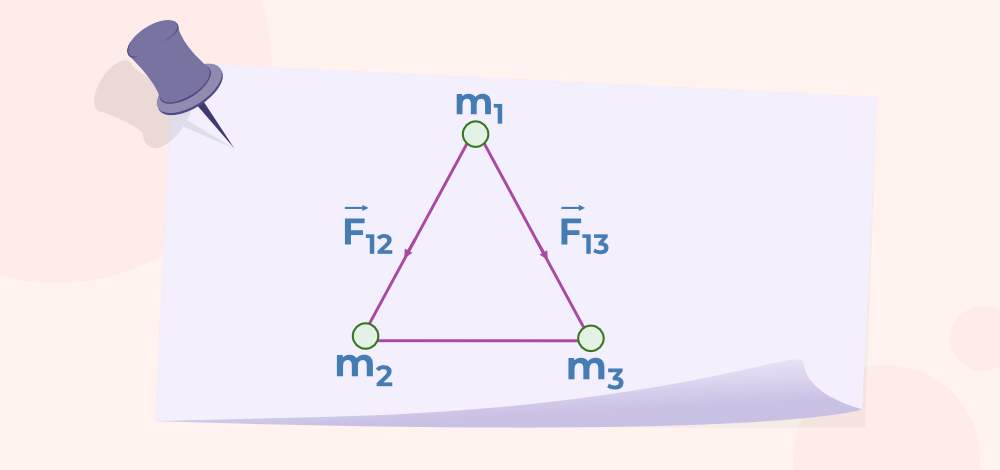Let F1 be the force acting on mass m which is at position 1.

By the Principle of Superposition of Gravitational Forces,F1 = Forces acting on mass m due to another mass m which is at position 2 + Forces acting on mass m due to another mass m which is at position 3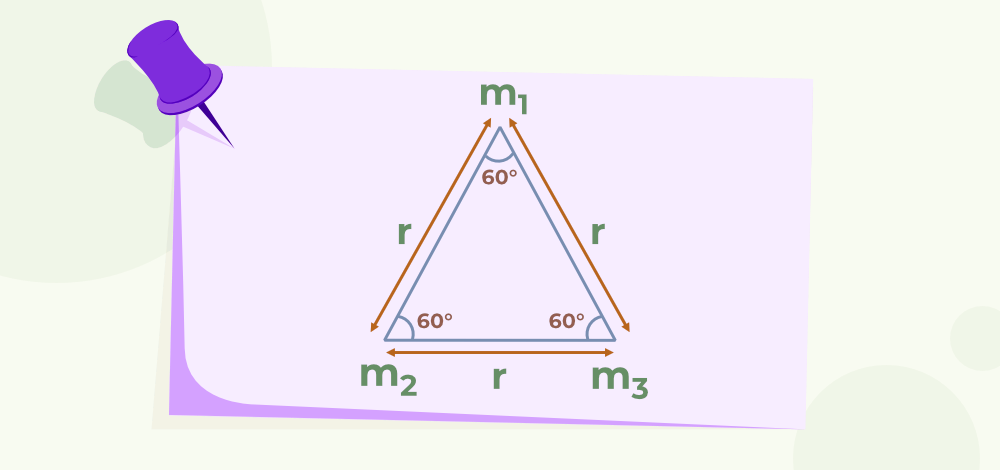Hereandmakes an angle of 60° to each other since they act along the sides of equilateral triangle.LetFres==Fres=√3f=√3×Gm2/r2

Thus the resultant force acting on mass m which is at position 1 and all the masses which are equal to m are placed in the vertices of the equilateral triangle having the length of its side is √3Gm2/r2.

Example 5: Three uniform spheres each having a mass M and radius a are kept in such a way that each touches the other two. Find the magnitude of the gravitational force on any of the spheres due to the other two spheres.

Solution:

Let A, B and C be the centers of three uniform spheres.Letbe the Force acting on C due to A andbe the force acting on C due to B

Let the total force acting on C due to A and B beBy the Principle of Superposition of Gravitational Forces,……(1)

On joining A,B and C, we get a equilateral triangle i.e, it makes an angle of 60° to each other.

Given radius of each sphere is a

so, AB = BC = CA = 2a

From Newton’s law of Gravitation,Here,From (1),==|F| =Thus the magnitude of the gravitational force on any of the sphere due to the other two spheres isExample 6: If Mercury, Venus, and the Sun are aligned in a right-angled triangle. Calculate the vector sum of forces on venus due to both mercury and Sun. What is the direction and magnitude of the resulting force?

Solution: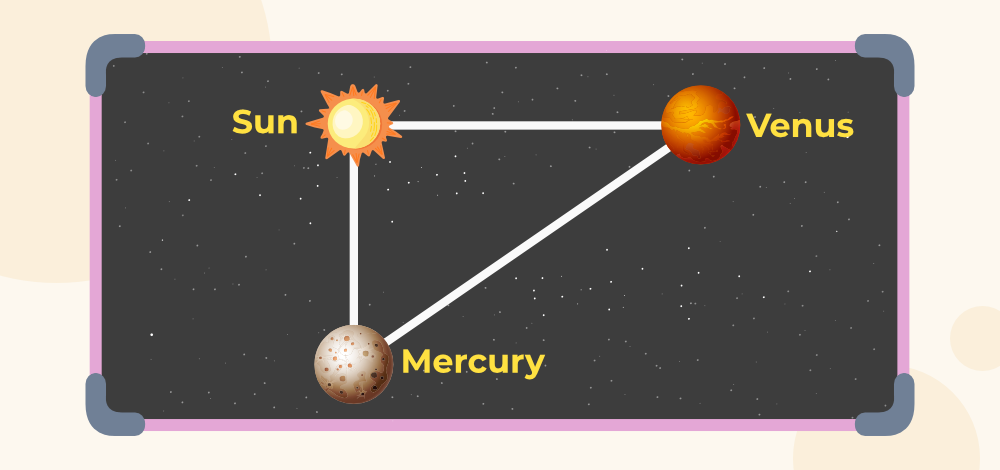Distance between Sun and Venus (rv) = 108×109 meters.

Distance between Sun and Mercury(rm) = 57.6×109 meters

Distance between Mercury and Venus (rmv)=rmv = 1.08×1011 meters.

Mass of Sun = 1.99×1030 kg

Mass of Mercury = 3.3×1023 kg

Mass of Venus = 4.87×1024 kg

Let Force acting on Venus due to Sun is FS

From Newton’s law of Gravitation,= 6.67×10-11×1.99×1030×4.87×1024/(108×109)^2

FS = 5.54×1022

Let Force acting on Venus due to Mercury is FM= (6.67 × 10-11 × 3.3 × 1023 × 4.87 ×1024)/(1.08×1011)2

FM = 9.19×1015 N

Here Force due to Sun is more than a million times greater than Force due to Mercury and so the net Force is mainly due to Sun and its magnitude is equal to Force acting due to Sun. And the direction of resulting Force similar to direction due to Sun.

## FAQs on Universal Law of Gravitation

### Q1: What is the Universal Law of Gravitation?

Newton’s Law of Universal Gravitation is also called the Universal Law of Gravitation. According to this law, every particle with mass m attracts every other particle which has mass and their force of attraction is directly proportional to the product of the masses of two objects and inversely proportional to the square of the distance between these objects.

### Q2: Why is the law of Gravitation called a Universal Law?

The law of gravitation is also called a Universal law because it is believed to work on all the objects in the universe.

### Q3: Who invented the Law of Gravitation?

The credit for inventing the Law of Gravitation goes to the famous mathematician and scientist Sir Issac Newton.

### Q4: What is the value of the Gravitational Constant G on the moon?

Gravitational Constant ‘G’ is a universal constant and hence the value of Gravitational Constant G on the moon is 6.67 × 10-11 Nm2 kg-2

### Q5: Is gravity force the same everywhere on Earth?

No, the gravity force is not the same everywhere on the Earth’s surface because the Earth’s surface is not uniform and its mass is not evenly distributed.

### Q6: Why doesn’t the moon fall into the Earth?

The moon does not fall into the Earth’s surface because it is maintained in its position by force of the Universal law of gravitation.

### Q7: What is the universal gravitational equation?

The universal gravitational equation is, F = G × (m1 × m2) / r2